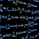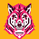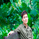13115 lượt xem
Thank You Richard Santos!!!

This is a great indicator guys.Its good for measuring divergence in an objective way.
The idea is to measure the high to high and the low to low (stock vs. indicator) with the low to high and high to low (stock vs. indicator). This is the basis of divergence.
I love this one.
I would also add you can draw angles on the indicator and the stocks. All in all a great indicator.

Richard Santos was nice enough to modify one of his scripts for this one. Credit goes to him.

You can also use this for measuring volatility when the lines narrow or widen.

```study(title='[RS]RSI Divergence V5')
length = input(5)

f_top_fractal(_src)=>_src < _src and _src < _src and _src > _src and _src > _src
f_bot_fractal(_src)=>_src > _src and _src > _src and _src < _src and _src < _src
f_fractalize(_src)=>f_top_fractal(_src) ? 1 : f_bot_fractal(_src) ? -1 : 0

rsi_high = rsi(high, length)
rsi_low = rsi(low, length)
fractal_top_rsi = f_fractalize(rsi_high) > 0 ? rsi_high : na
fractal_bot_rsi = f_fractalize(rsi_low) < 0 ? rsi_low : na

rsi_high_prev = valuewhen(fractal_top_rsi, rsi_high, 1)
rsi_high_price = valuewhen(fractal_top_rsi, high, 1)
rsi_low_prev = valuewhen(fractal_bot_rsi, rsi_low, 1)
rsi_low_price = valuewhen(fractal_bot_rsi, low, 1)

regular_bearish_div = fractal_top_rsi and high > rsi_high_price and rsi_high < rsi_high_prev
hidden_bearish_div = fractal_top_rsi and high < rsi_high_price and rsi_high > rsi_high_prev
regular_bullish_div = fractal_bot_rsi and low < rsi_low_price and rsi_low > rsi_low_prev
hidden_bullish_div = fractal_bot_rsi and low > rsi_low_price and rsi_low < rsi_low_prev

plot(title='RSI High', series=rsi_high, color=gray)
plot(title='RSI Low', series=rsi_low, color=gray)
plot(title='RSI H F', series=fractal_top_rsi, color=black, offset=-2)
plot(title='RSI L F', series=fractal_bot_rsi, color=black, offset=-2)
plot(title='RSI H D', series=fractal_top_rsi, style=circles, color=regular_bearish_div or hidden_bearish_div ? maroon : gray, linewidth=3, offset=-2)
plot(title='RSI L D', series=fractal_bot_rsi, style=circles, color=regular_bullish_div or hidden_bullish_div ? green : gray, linewidth=3, offset=-2)

plotshape(title='+RBD', series=regular_bearish_div ? rsi_high : na, text='Regular', style=shape.labeldown, location=location.absolute, color=maroon, textcolor=white, offset=-2)
plotshape(title='+HBD', series=hidden_bearish_div ? rsi_high : na, text='hidden', style=shape.labeldown, location=location.absolute, color=maroon, textcolor=white, offset=-2)
plotshape(title='-RBD', series=regular_bullish_div ? rsi_low : na, text='Regular', style=shape.labelup, location=location.absolute, color=green, textcolor=white, offset=-2)
plotshape(title='-HBD', series=hidden_bullish_div ? rsi_low : na, text='hidden', style=shape.labelup, location=location.absolute, color=green, textcolor=white, offset=-2)```

## Bình luận@SpreadEagle71 Thanks for the indicator. How can I find the angle of the divergence between price and RSI? I want to find the ones more than 30 degrees for example. Thanks in advance.
Phản hồiLeant so much with this script. Thanks for sharing
Phản hồican you combine
plotshape(title='+RBD', series=regular_bearish_div ? rsi_high : na, text='Regular', style=shape.labeldown, location=location.absolute, color=maroon, textcolor=white, offset=-2)
plotshape(title='+HBD', series=hidden_bearish_div ? rsi_high : na, text='hidden', style=shape.labeldown, location=location.absolute, color=maroon, textcolor=white, offset=-2)
plotshape(title='-RBD', series=regular_bullish_div ? rsi_low : na, text='Regular', style=shape.labelup, location=location.absolute, color=green, textcolor=white, offset=-2)
plotshape(title='-HBD', series=hidden_bullish_div ? rsi_low : na, text='hidden', style=shape.labelup, location=location.absolute, color=green, textcolor=white, offset=-2)

in one plotshape so i can put them all on one alarm ?
Phản hồiHey, this looks great. Is there any way to add an alarm for when the two indicators appear? Would appreciate your help, thanks
Phản hồiThank you , do you have indication for RSI reversals as well?
Phản hồi